Reach Us+44-1904-929220
Modeling of Three-Dimensional Soft Tissue Deformation with Dynamic Nonlinear Finite Element | OMICS International
Journal of Applied Mechanical Engineering
All submissions of the EM system will be redirected to Online Manuscript Submission System. Authors are requested to submit articles directly to Online Manuscript Submission System of respective journal.

Modeling of Three-Dimensional Soft Tissue Deformation with Dynamic Nonlinear Finite Element

Bahwini T1*, Zhong Y1, Gu C1 and Smith J2

1School of Engineering, RMIT University, Melbourne, Australia

2Department of Surgery, School of Clinical Sciences at Monash Health, Monash University, Clayton, Australia

*Corresponding Author:
Bahwini T
School of Engineering, RMIT University
Melbourne, Australia
Tel: +613 9925 6018
E-mail: [email protected]

Received Date: September 26, 2016; Accepted Date: October 18, 2016; Published Date: October 22, 2016

Citation: Bahwini T, Zhong Y, Gu C, Smith J (2016) Modeling of Three-Dimensional Soft Tissue Deformation with Dynamic Nonlinear Finite Element. J Appl Mech Eng 5:237. doi: 10.4172/2168-9873.1000237

Copyright: © 2016 Bahwini T, et al. This is an open-access article distributed under the terms of the Creative Commons Attribution License, which permits unrestricted use, distribution, and reproduction in any medium, provided the original author and source are credited.

Visit for more related articles at Journal of Applied Mechanical Engineering

Abstract

Soft tissue deformation is a significant part of surgical simulation. This paper presents a dynamic nonlinear finite element method for modeling of soft tissue deformation. This method models large-range deformation via the secondorder Piola-Kirchhoff stress. It condenses the stiffness matrix to reduce the degrees of freedom of the entire soft body at each node for every time step to improve the computational performance. Simulations and comparison analysis show that the proposed method can predict the nonlinear behaviors of soft tissues and requires a small amount of time.

Keywords

Surgical simulation; Soft tissue deformation; Finite element; Second-order Piola-Kirchhoff Stress; Large-range deformation

Introduction

Soft tissue deformation plays a vital role in surgical simulation [1- 3]. Surgical simulation requires the mechanical interaction between soft tissues and surgical tools be realistic and in real-time . However, due to the complexity of soft tissues, it is difficult to achieve both conflict requirements, and realistic modeling of soft tissue deformation in real time is still a challenging research problem .

The mass-spring model and finite element method (FEM) are the most common modeling methods for soft tissue deformation . The mass-spring model uses springs connected masses to carry out soft tissue deformation. It is simple in computation and easy to implement, but lacks the physical accuracy. The FEM is the exact opposite to the mass-spring model. It carries out soft tissue deformation based on rigorous laws in continuum mechanics, leading to high accuracy for modelling. However, it is expensive in computation. Due to the complexity in computation, the existing FEM models are mainly based on linear elasticity.

This paper presents a nonlinear FEM for modeling of soft tissue deformation. This method uses the second-order Piola-Kirchhoff stress for modeling of nonlinear soft tissue behaviors. The technique of matrix condensation is also developed to improve the computational performance by reducing the degree of freedom. The rest of the paper is organized as follows: After the literature survey in section 2, section 3 details the proposed method for modelling of soft tissue deformation. Section 4 evaluates the performance of the proposed method. Finally, Section 5 concludes the paper and discusses about the future work.

Literature Review

There have been significant research efforts in soft tissue modeling for surgical simulation and robotic-assisted surgery, and development of virtual surgical schemes for education . Various FEMs have been developed for linear elastic 2D and 3D simulations [7-10]. DiMaio and Salcudean established force distribution over the needle shaft, and developed a 2D finite element simulation using a linear electrostatic material model to measure the tissue deformation path in a phantom tissue . They also applied fast low-rank matrix updates to achieve the real-time contact simulation . Alterovitz et al. developed a dynamic system for needle insertion using the mass-spring model. This system can simulate the needle insertion process with improved accuracy and computational performance .

Okamura et al. developed an empirical force model for soft tissue deformation and penetration, where the needle forces are considered to be a combination of the stiffness force, friction force and cutting force . Webster et al. studied the motion of needle insertion with the use of a bevel-tip needle . Misra et al. reported a mechanics-based method for steering of needle motion by exploring the connections at the tip and the overall bending of the needle with consideration of material properties and the needle tip geometry . Mahvash and Dupont extended Misra’s work and developed a dynamic model for characterizing rupture events, showing that the rupture force would decrease when the needle insertion velocity increases . Wang and Hirai developed a dynamic model of needle-tissue interaction by considering needle insertion as a mixture of contact, rupture and friction forces . They also applied a local constraint method to avoid re-meshing, which is generally needed due to the collision between discontinuous finite element structures and continuous needle movement.

In general, the existing FEM methods are mainly dominated by linear elasticity to reduce the computational cost, thus unsuited to handle nonlinear elastic behaviors of soft tissues.

Methodology

Proposed 3D dynamic nonlinear soft tissue model

Modeling of soft tissue behaviors depends on material properties. The mechanical behavior of an object, which results from the object internal structure, can be characterized using constitutive relations. Consider an isotropic and homogenous object with nonlinear elastic behavior. For large deformation, the strain is described as,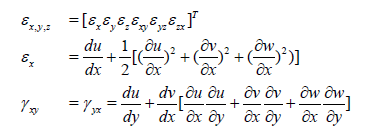(1)

where εx is the normal strain, and γxy = γyx is the shear strain. The other terms of the strain can be defined similarly.

In order to describe the large deformation in a global form in terms of nonlinear quadratic strain, let us consider a fixed global coordinate system for the entire simulation. Therefore,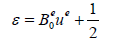(2)

Where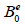and ue represent the linear displacement differentiation matrix in size of 6 × 3 and the nodal displacement in size of 3 × × 1, respectively.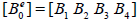is for each element in the tetrahedron. The second item on the left side represents the nonlinear displacement differentiation matrix and the nodal displacement, where A is a 6 × 9 nonlinear matrix, and Θ is a 9 × 1 matrix with three-dimensional identity ( I3 ).

Under the assumption of nonlinear elastic material, the stress σ and the strain ε are in a non-linear relationship, which can be defined by Hooke’s law as,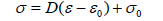(3)

where σ0 and σ are the stresses at times t0 and tn+1 respectively, D is the elasticity matrix,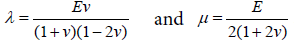are the material parameters, and ε0 and ε are the strains at times t0 and tn+1. E and v denote the Young’s modulus and Poisson’s ratio, respectively.

Constitutive relation for nonlinear FEM

The strain energy density function (SEDF) is a common method to describe material behavior. The deformation of a physical object can be characterized by the deformation gradient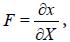where X and x are the original and the deformed configurations, respectively . In the 3-D nonlinear case, the second-order gradient tensor (internal force) at a given point inside a material has nine strain components, which define the state of stress and strain at the point for the deformation configuration. The material will be hyper elastic when such SEDF exists, from which the stress components can also be derived. Let W be the strain energy per unit volume of the tissue. In a hyper-elastic material, when SEDF (which is derived from the Cauchy stress tensor in the material because of deformation) is known, it can be obtained from the second Piola-Kirchhoff stress,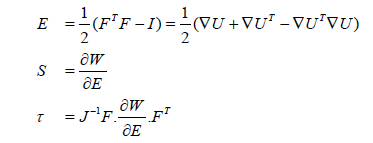(4)

where E is the Green (nonlinear) strain tensor, S is the second Piola- Kirchhoff stress, U= x-X is the displacement vector, I is the identity tensor, J is the determinant of F, and τ is the Cauchy stress tensor. For a hyper-elastic material, the SEDF can be derived from Hooke’s law as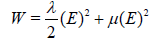(5)

Element stiffness matrix

The global deformation is large and involves the entire body. This type of deformation is essential to surgical simulation, and can be mathematically applied to any large motion and deformation. The nonlinear stiffness is,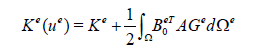(6)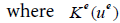is the local nonlinear stiffness matrix, which is dependent on the displacement ue. A and Ge are the nonlinear deferential matrices. Equation (10) can be further simplified as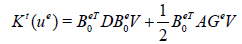(7)

where Kt (ue ) is the nonlinear strain incremental stiffness matrices at time t, and V is the volume of the integral element.

Global equation to nonlinear finite element modelling

The element behavior is characterized by the partial differential equation governing the motion of the material points of a continuum, resulting in the following discrete system differential equation (equation of motion):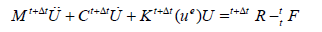(8)

Where U is the displacement vector at a node,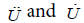are the acceleration and velocity at time t +Δt, F is the external force vector at time t, M and C are the time-dependent mass and damping matrices, and R is the external load applied at the nodal at time t + Δt .

Static condensation method

The static condensation method is employed to reduce the number of degrees of freedom for the element, that is, condense out the internal nodes. This method is to determine a part of the solution to solve the total finite element system equilibrium equations prior to assembling the structure matrices K and U based on the system boundary, leading to reduced computations. The stiffness matrix and corresponding displacement and force vector of the element under consideration can be decomposed into the form,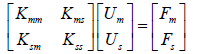(9)

where m and s are the degrees of freedom of master (to be returned) and slave nodes (to be condensed out), and Um and Us are the desired displacements of the master and slave nodes, and Fm is the load vector.

From (13), we have the condition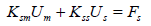(10)

which can be used to eliminate Us. From (14) we can obtain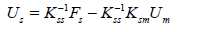(11)

Substituting (15) into (13) yields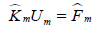(12)

where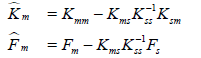(13)

The new stiffness matrix Km in the reduced form (16) is obviously denser than the stiffness matrix in the original form (14). This means that the condensed method is mathematically equivalent to the volumetric FEM, keeping the volume characteristic in the solution, but only at the computational expense of the surface FEM.

Model dynamics

The dynamics of the nonlinear FEM is achieved using an implicit Lagrangian formulation. The implicit Lagrangian formulation can be solved with the Newmark’s method, leading to unconditionally stable solutions.

By linearizing the motion (12), the nonlinear FEM at each time step after the initial calculation can be described as follows:

1) Calculate effective loads at time t + Δt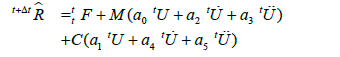(14)

2) Solve for displacements at time t + Δt.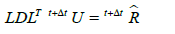(15)

3) Calculate the accelerations and velocities at time t + Δt: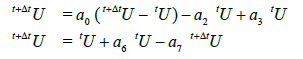(16)

Where δ=0.5 and α=0.25 are the parameters to obtain the accuracy and stability, a0, a1…a7 are the coefficient parameters, and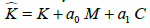is the effective stiffness matrix. Using the defactorisation (Skyline) method,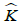can be reduced to an upper triangular form, from which the unknown displacement U can be calculated by back-substitution.

Deformation region selection

With the model dynamics, the topology structure of the deformation for soft tissue modeling using FEM will be reformed and subsequently the stiffness matrix of the deform structure will be updated. Therefore, in order to reduce the computational time and cost, the minimum faces of the model mesh at the deformation region must be determined.

Strictly speaking, a set of triangles from the mesh, which are close to the surgical tool need to be selected. Generally, the selection of the minimum deformation region is according to the interaction between the surgical tool and soft tissues, which is detected based on the information of faces, edges and vertices. Figure 1 shows the three cases for the determination of the minimum deformation region. The interaction process between the surgical tool and soft tissues is highlighted in red and the minimum deformation area is displayed in green. The minimum deformation region is determined based on the following interaction configurations: (a) Vertex: six triangles are adjusted; (b) Edge: two triangles are adjusted; and (c) Face: one triangle is adjusted.

Figure 1: Different interaction cases between the surgical tool and soft tissues (a) Vertex (b) Edge and (c) Face .

Implementation and result

The prototype system for simulation of soft tissue deformation with the proposed FEM was implemented using Java3D on a PC with Intel Core i7 MacBook Pro (13-inch, Early 2011) at 2.7 GHz with 16 GB 1333 MHz DDR3 RAM memory and Intel HD Graphics 3000 512 MB. Simulations were conducted to investigate the performance of the proposed nonlinear FEM. The values of materials parameters were set according to those reported in .

Trials on the interaction between a surgical needle and soft tissues were conducted by the proposed nonlinear FEM. Different shapes of tetrahedron volume models such as the cube, human liver and kidney were tested. Table 1 shows the element numbers after condensation and the average iteration computational times. For example, for the cubic volume model which is made up of 953 elements, it is condensed to 266 tetrahedron elements, and the average time for one iteration of deformation is around 0.174 seconds. As the visual refresh rate should be more than 25 frames per second. Yin and Goulette proposed FEM is able to provide real-time visual feedback [19,20]. Figure 2 shows the deformations of the cubic model under a tensile and compression force, respectively. Figures 3 and 4 show more deformation results on the cubic model. Figure 5 illustrates the deformations of the virtual human liver and kidney models.

Figure 2: Cubic modeling (a) wireframe (b) shade (c) and (d) deformed force (under a tensile and compression force, respectively).

Object
Test
Number of elements Condensation Average of one iteration time costs (Second)
Condensed elements Dynamic
Cubic 953 266  0.174
Liver 4094 873  0.881
Kidney 11494 2188  7.142

Table 1: Matrix condensation and time performance.

To further evaluate the performance of the proposed FEM, we further compared the simulation results with experimental data reported in the literature . The simulation results in terms of the relationships between stress and strain as well as force and displacement are shown in Figures 6 and 7, while the corresponding experimental data are shown in Figure 8. It is obvious that the proposed FEM demonstrates the nonlinear deformation behavior remarkably. Therefore, the proposed FEM can achieve large deformations via the nonlinear relationship.

Conclusion

This paper presents a dynamic nonlinear FEM for simulating soft tissue deformation for surgical simulation. This method carries out soft tissue deformation using the second-order Piola-Kirchhoff stress. The model dynamics is conducted based on the implicit Lagrangian formulation to define the nonlinear constitutive relationships for large deformations. The Newmark’s method under the assumption of isotropic and homogenous materials is established. Moreover, a technique of matrix condensation based on continuum mechanics of solid is also developed to reduce the number of the degrees of freedom for each element.

Future work will focus on the improvement and application of the proposed FEM for surgical simulation. The proposed FEM will be integrated with a haptic device for achieving force feedback for surgical simulation. It is expected to establish the methods of haptic modeling and rendering for soft tissue deformation with real-time haptic feedback in real time. The proposed FEM will also be applied to model the typical surgical procedure of needle insertion and further develop a surgical simulation system for training and procedure planning of needle insertion.

Acknowledgment

This work was supported by Ministry of Education in Saudi Arabia for financial support for the first author on his research.

References

Select your language of interest to view the total content in your interested language

Article Usage

• Total views: 9271
• [From(publication date):
December-2016 - Jun 26, 2019]
• Breakdown by view type
• HTML page views : 9072Can't read the image? click here to refresh